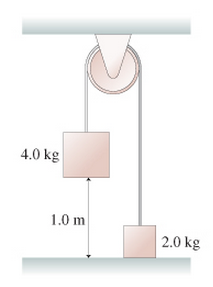# Problem: The two blocks in the figure(Figure 1) are connected by a massless rope that passes over a pulley. The pulley is 12cm in diameter and has a mass of 2.7kg. As the pulley turns, friction at the axle exerts a torque of magnitude 0.50N/m.If the blocks are released from rest, how long does it take the 4.0 kg block to reach the floor?

###### FREE Expert Solution

Torque equations:

$\overline{){\mathbit{\Sigma }}{\mathbit{\tau }}{\mathbf{=}}{\mathbit{\alpha }}{\mathbit{I}}}$ where I is the moment of inertia.

$\overline{){\mathbit{\tau }}{\mathbf{=}}{\mathbit{r}}{\mathbit{F}}}$

Relationship between linear and angular acceleration:

$\overline{){\mathbf{\alpha }}{\mathbf{=}}\frac{\mathbf{a}}{\mathbf{r}}}$

Moment of inertia of a disk(pulley):

$\overline{){\mathbf{I}}{\mathbf{=}}\frac{\mathbf{1}}{\mathbf{2}}{\mathbf{m}}{{\mathbf{r}}}^{{\mathbf{2}}}}$

Uniform accelerated motion (UAM) equations:

We'll determine the value of the linear acceleration and apply kinematics to solve for t.

Take the T1 to be the tension in left rope and T2 to be the tension in the right rope. T1 creates counterclockwise torque on the pulley while T2 creates clockwise torque on the pulley. The pulley rotates counterclockwise, meaning that the torque due to friction is clockwise.

93% (378 ratings)###### Problem Details

The two blocks in the figure(Figure 1) are connected by a massless rope that passes over a pulley. The pulley is 12cm in diameter and has a mass of 2.7kg. As the pulley turns, friction at the axle exerts a torque of magnitude 0.50N/m.

If the blocks are released from rest, how long does it take the 4.0 kg block to reach the floor?